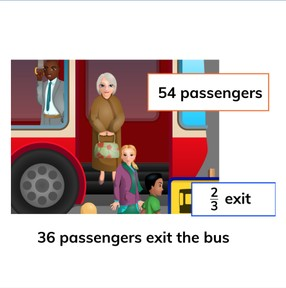Calculating with fractions

# Calculating with fractions

I can calculate a portion, a missing portion, the fraction and/or the total.8,000 schools use Gynzy92,000 teachers use Gynzy1,600,000 students use Gynzy

## General

Students can calculate a portion, a missing portion, the fraction and/or the total of a set using fractions.

## Common core standard(s)

CCSS.MATH.CONTENT.5.NF.B.4

## Relevance

It is important to learn this so you can calculate how much is in a portion, what a missing portion is, the fraction or the total of a set.

## Introduction

Ask students to simplify the given fraction as far as possible.

## Development

Discuss the importance of being able to calculate with fractions. Refresh the parts of a fraction by showing and naming the numerator and denominator. Then refresh the instructions on how to calculate a portion, how to calculate the missing portion, how to calculate the fraction and how to calculate the total, all using fractions. Show students how to calculate the problem with cows. You first calculate what 1//3 of the cows is. You divide the total by 3. 15 ÷ 3 = 5.
By subtracting this number from the total you discover the missing portion. You can then determine the fraction. Do the problem with the fish together. You can show the steps by dragging the covers from the steps on the interactive whiteboard. Check with the next exercises that students can calculate with fractions.

Check that students can calculate with fraction by asking the following question:
- Which steps do you use to calculate with fractions?

## Guided practice

Students first practice with an exercise in which they must determine the missing portion. Next they must drag the correct numbers to the labelled tabels and then they must calculate from a fraction.

## Closing

Discuss with students the importance of being able to calculate with fractions. To close you can ask students to work in pairs to determine which numbers belong in which column as given on the interactive whiteboard.

## Teaching tips

Students who have difficulty can be supported by first practicing with problems that only ask them to solve 1 step. So only solving for a part, only solving for the fraction, only solving for the missing portion, or only solving to find the total. Then you can continue to practice the element they have the most difficulty with.

## Instruction materials

Optional: During the lesson "real" or tangible materials can be used to split into fractions, like a pie or cake.

### The online teaching platform for interactive whiteboards and displays in schools

• Save time building lessons

• Manage the classroom more efficiently

• Increase student engagement